# Combining Multiply Occurring Fields and Multiple Record Types

You can have two types of descendant segments in a single fixed-format sequential, VSAM, or ISAM data source:

• Descendant segments consisting of multiply occurring fields.
• Additional descendant segments consisting of multiple record types.

## Describing a Multiply Occurring Field and Multiple Record Types

In the data structure shown below, the first record (of type 01) contains several different sequences of repeating fields, all of which must be described as descendant segments with an OCCURS attribute. The data source also contains two separate records, of types 02 and 03, which contain information that is related to that in record type 01.

The relationship between the records of various types is expressed as parent-child relationships. The children that contain record types 02 and 03 do not have an OCCURS attribute. They are distinguished from their parent by the field declaration where field=RECTYPE.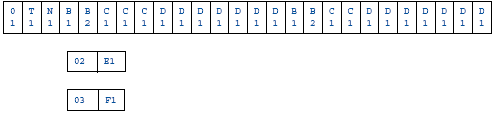The description for this data source is:

```FILENAME = EXAMPLE1, SUFFIX = FIX,\$
SEGNAME = A, SEGTYPE=S0,\$
FIELDNAME = RECTYPE   ,ALIAS = 01   ,USAGE = A2   ,ACTUAL = A2 ,\$
FIELDNAME = T1        ,ALIAS =      ,USAGE = A2   ,ACTUAL = A1 ,\$
FIELDNAME = N1        ,ALIAS =      ,USAGE = A1   ,ACTUAL = A1 ,\$
SEGNAME = B, PARENT = A, OCCURS = VARIABLE, SEGTYPE=S0,\$
FIELDNAME = B1        ,ALIAS =      ,USAGE = I2   ,ACTUAL = I2 ,\$
FIELDNAME = B2        ,ALIAS =      ,USAGE = I2   ,ACTUAL = I2 ,\$
SEGNAME = C, PARENT = B, OCCURS = B1, SEGTYPE=S0,\$
FIELDNAME = C1        ,ALIAS =      ,USAGE = A1   ,ACTUAL = A1 ,\$
SEGNAME = D, PARENT = B, OCCURS = 7,  SEGTYPE=S0,\$
FIELDNAME = D1        ,ALIAS =      ,USAGE = A1   ,ACTUAL = A1 ,\$
SEGNAME = E, PARENT = A, SEGTYPE=S0,\$
FIELDNAME = RECTYPE   ,ALIAS = 02   ,USAGE = A2   ,ACTUAL = A2 ,\$
FIELDNAME = E1        ,ALIAS =      ,USAGE = A1   ,ACTUAL = A1 ,\$
SEGNAME = F, PARENT = E, SEGTYPE=S0,\$
FIELDNAME = RECTYPE   ,ALIAS = 03   ,USAGE = A2   ,ACTUAL = A2 ,\$
FIELDNAME = F1        ,ALIAS =      ,USAGE = A1   ,ACTUAL = A1 ,\$```

It produces the following data structure: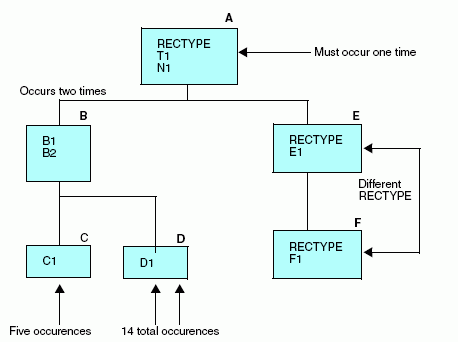Segments A, B, C, and D all belong to the same record type. Segments E and F each are stored as separate record types.

Note:
• Segments A, E, and F are different records that are related through their record types. The record type attribute consists of certain prescribed values, and is stored in a fixed location in the records. Records are expected to be retrieved in a given order. If the first record does not have a RECTYPE of 01, the record is considered to be a child without a parent. The next record can have a RECTYPE of either 01 (in which case, the first record is considered to have no descendants except the OCCURS descendants) or 02. A record with a RECTYPE of 03 can follow only a record with a RECTYPE of 02 (its parent) or another 03.
• The OCCURS descendants all belong to the record whose RECTYPE is 01. (This is not a necessary condition. Records of any type can have OCCURS descendants.) Note that the OCCURS=VARIABLE segment, Segment B, is the right-most segment within its own record type. If you look at the data structure, the pattern that makes up Segment B and its descendants (the repetition of fields B1, B2, C1, and D1) extends from the first mention of fields B1 and B2 to the end of the record.
• Although fields C1 and D1 appear in separate segments, they are actually part of the repeating pattern that makes up the OCCURS=VARIABLE segment. Since they occur multiple times within Segment B, they are each assigned to their own descendant segment. The number of times field C1 occurs depends on the value of field B2. In the example, the first value of field B2 is 3. The second, 2. Field D1 occurs a fixed number of times, 7.

## Describing a VSAM Repeating Group With RECTYPEs

Suppose you want to describe a data source that, schematically, looks like this:

 `A RECTYPE B C RECTYPE B C` `A RECTYPE D RECTYPE D`

You must describe three segments in your Master File, with A as the root segment, and segments for B, C, and D as two descendant OCCURS segments for A.

The following diagram illustrates these segments.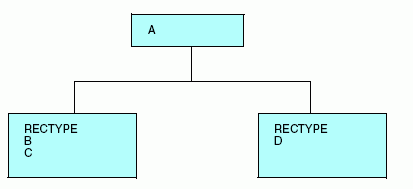Each of the two descendant OCCURS segments in this example depends on the RECTYPE indicator that appears for each occurrence.

All the rules of syntax for using RECTYPE fields and OCCURS segments also apply to RECTYPEs within OCCURS segments.

Since each OCCURS segment depends on the RECTYPE indicator for its evaluation, the RECTYPE must appear at the start of the OCCURS segment. This enables you to describe complex data sources, including those with nested and parallel repeating groups that depend on RECTYPEs.

### Example: Describing a VSAM Repeating Group With RECTYPEs

In this example, B/C, and D represent a nested repeating group, and E represents a parallel repeating group.

 `A` `RECTYPE B C ` `RECTYPE D` `RECTYPE E` `RECTYPE E`
```FILENAME=SAMPLE,SUFFIX=VSAM,\$
SEGNAME=ROOT,SEGTYPE=S0,\$
GROUP=GRPKEY      ,ALIAS=KEY ,USAGE=A8 ,ACTUAL=A8 ,\$
FIELD=FLD000     ,E00       ,A08      ,A08       ,\$
FIELD=A_DATA     ,E01       ,A02      ,A02       ,\$
SEGNAME=SEG001,PARENT=ROOT,OCCURS=VARIABLE,SEGTYPE=S0  ,\$
FIELD=RECTYPE     ,A01       ,A01      ,ACCEPT=B OR C ,\$
FIELD=B_OR_C_DATA ,E02       ,A08      ,A08       ,\$
SEGNAME=SEG002,PARENT=SEG001,OCCURS=VARIABLE,SEGTYPE=S0,\$
FIELD=RECTYPE     ,D         ,A01      ,A01       ,\$
FIELD=D_DATA      ,E03       ,A07      ,A07       ,\$
SEGNAME=SEG003,PARENT=ROOT,OCCURS=VARIABLE,SEGTYPE=S0  ,\$
FIELD=RECTYPE     ,E         ,A01      ,A01       ,\$
FIELD=E_DATA      ,E04       ,A06      ,A06       ,\$```

## Describing a Repeating Group Using MAPFIELD

In another combination of record indicator and OCCURS, a record contains a record indicator that is followed by a repeating group. In this case, the record indicator is in the fixed portion of the record, not in each occurrence. Schematically, the record appears like this: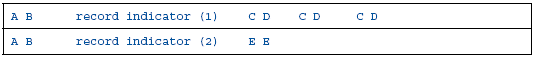The first record contains header information, values for A and B, followed by an OCCURS segment of C and D that was identified by its preceding record indicator. The second record has a different record indicator and contains a different repeating group, this time for E.

The following diagram illustrates this relationship: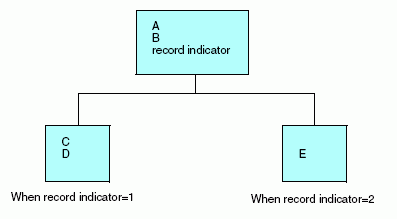Since the OCCURS segments are identified by the record indicator rather than the parentA/B segment, you must use the keyword MAPFIELD. MAPFIELD identifies a field in the same way as RECTYPE, but since each OCCURS segment has its own value for MAPFIELD, the value of MAPFIELD is associated with each OCCURS segment by means of a complementary field named MAPVALUE.

The following diagram illustrates this relationship: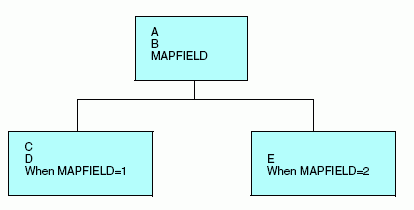MAPFIELD is assigned as the ALIAS of the field that is the record indicator. It may have any name.

### Syntax: How to Describe a Repeating Group With MAPFIELD

`FIELD = name, ALIAS = MAPFIELD, USAGE = format, ACTUAL = format,\$`

where:

name

Is the name you choose to provide for this field.

ALIAS

MAPFIELD is assigned as the alias of the field that is the RECTYPE indicator.

USAGE

Follows the usual field format.

ACTUAL

Follows the usual field format.

The descendant segment values depend on the value of the MAPFIELD. They are described as separate segments, one for each possible value of MAPFIELD, and all descending from the segment that has the MAPFIELD. A special field, MAPVALUE, is described as the last field in these descendant segments after the ORDER field, if one has been used. The actual MAPFIELD value is supplied as the ALIAS of MAPVALUE.

### Syntax: How to Use MAPFIELD for a Descendant Repeating Segment in a Repeating Group

```FIELD = MAPVALUE, ALIAS = alias, USAGE = format, ACTUAL = format,
ACCEPT = {list|range} ,\$```

where:

MAPVALUE

Indicates that the segment depends on a MAPFIELD in its parent segment.

alias

Is the primary MAPFIELD value, if an ACCEPT list is not specified. If there is an ACCEPT list, this can be any value.

USAGE

Is the same format as the MAPFIELD format in the parent segment.

ACTUAL

Is the same format as the MAPFIELD format in the parent segment.

list

Is the list of one or more lines of specified MAPFIELD values for records that have the same segment layout. The maximum number of characters allowed in the list is 255. Each item in the list must be separated by either a blank or the keyword OR. If the list contains embedded blanks or commas, it must be enclosed within single quotation marks ('). The list may contain a single MAPFIELD value.

For example:

```FIELDNAME = MAPVALUE, ALIAS = A, USAGE = A1, ACTUAL = A1,
ACCEPT = A OR B OR C,\$```
range

Is a range of one or more lines of MAPFIELD values for records that have the same segment layout. The maximum number of characters allowed in the range is 255. If the range contains embedded blanks or commas, it must be enclosed in single quotation marks (').

To specify a range of values, include the lowest value, the keyword TO, and the highest value, in that order.

#### Example: Using MAPFIELD and MAPVALUE

Using the sample data source at the beginning of this section, the Master File for this data source looks like this:

```FILENAME=EXAMPLE,SUFFIX=FIX,\$
SEGNAME=ROOT,SEGTYPE=S0,\$
FIELD =A,              ,A14   ,A14 ,\$
FIELD =B,              ,A10   ,A10 ,\$
FIELD =FLAG ,MAPFIELD  ,A01   ,A01 ,\$
SEGNAME=SEG001,PARENT=ROOT,OCCURS=VARIABLE,SEGTYPE=S0  ,\$
FIELD =C,              ,A05   ,A05 ,\$
FIELD =D,              ,A07   ,A07 ,\$
FIELD =MAPVALUE ,1     ,A01   ,A01 ,\$
SEGNAME=SEG002,PARENT=ROOT,OCCURS=VARIABLE,SEGTYPE=S0  ,\$
FIELD =E,              ,D12.2 ,D8  ,\$
FIELD =MAPVALUE ,2     ,A01   ,A01 ,\$```

Note: MAPFIELD can only exist on an OCCURS segment that has not been re-mapped. This means that the segment definition cannot contain POSITION=fieldname.

MAPFIELD and MAPVALUE may be used with SUFFIX=FIX and SUFFIX=VSAM data sources.

 WebFOCUS